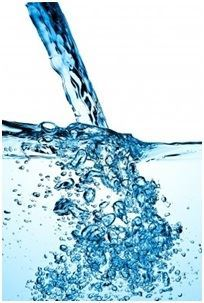# Flow Rate Formula

Flow Rate Formula

As we all know, liquids flow. One can say how slow or fast it flows. But can someone tell at what rate Fluid flows? To compute that let’s study the flow rate. The flow rate of a liquid is how much fluid passes through an area in a particular time. Flow rate can be articulated in either terms of velocity and cross-sectional area, or time and volume. As liquids are incompressible, the rate of flow into an area must be equivalent to the rate of flow out of an area. This is identified as the equation of continuity.Flow rate is the quantity of fluid flowing in the specified time. It is expressed in liters per meter (lpm) or gallons per meter (gpm) . It is articulated as

Where,
the flow area is A and

the flow velocity is v.

Flow rate can also be articulated as in a given time (t) the capacity of fluid stored (C) . It is also articulated as

Where,
the capacity of fluid stored is C and
the time taken to flow is t

Flow rate formula has extensive applications in fluid dynamics to compute the velocity, area or flow rate.

Flow Rate Solved Examples

Provided underneath are the questions grounded on flow rate which may be useful for you.

Problem 1: Compute the flow rate of fluid if it is moving with the velocity of 20 m/s through a tube of diameter 0.03 m.